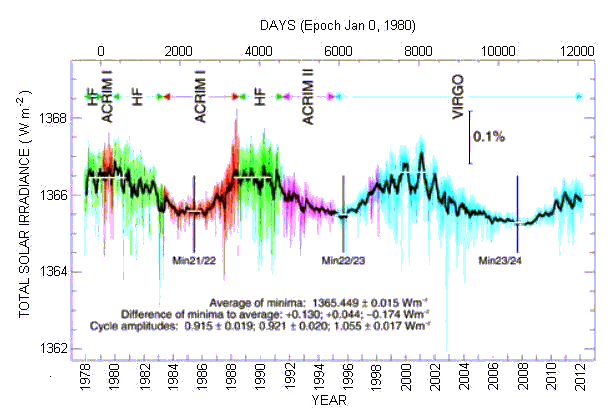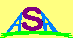### THE SOLAR CONSTANT & SOLAR VARIABILITY

The luminosity of the Sun is about 3.86 x 1026 watts. This is the total power radiated out into space by the Sun. Most of this radiation is in the visible and infrared part of the electromagnetic spectrum, with less the 1% emitted in the radio, UV and X-ray spectral bands. This energy is also radiated isotropically, that is, uniformly into space, independent of direction.

Because the Sun is about 150 million kilometres from the Earth, and because Earth is about 6380 km in radius, only 0.000000045% of this power is intercepted by our planet. This still amounts to a massive 1.75 x 1017 watts. For the purposes of solar energy capture, we normally talk about the amount of power in sunlight passing through one square metre at the Earth's distance from the Sun (and that one square metre is assumed to be at normal incidence, or 'face on' to the direction from which the sunlight is coming). This power is termed the solar constant, and is approximately 1370 watts per square metre (W m-2).

Now this solar constant is actually not a true constant. It varies by +/- 3% because of the Earth's slightly elliptical orbit around the Sun, being larger when the Earth is at perihelion (currently the first week in January) and smaller when the Earth is at aphelion (currently the first week in July). Some people, when talking about the solar constant, correct for this distance variation, and refer to the solar constant as the power per unit area received at the average Earth-Sun distance (this is called the Astronomical Unit or AU and has the value 149.59787066 million kilometres).

Even when we correct for the changing Earth-Sun distance we still find that there is a small variation in the solar constant, and this is due to a variation in the intrinsic luminosity of the Sun itself. This variation has been measured by radiometers aboard several satellites since the late 1970's and is shown in the graph below. This is a composite graph produced by the World Radiation Centre and shows that our Sun is really a (slightly) variable star.Australian Space Academy